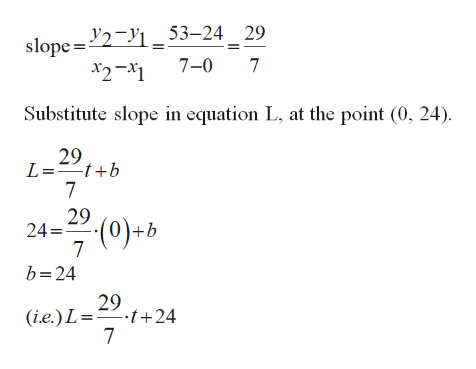# Newborn blue whales have a length of about 24 feet and weight 3 tons. These young whales are breast-fed for 7 months, and when they stop, they have a length of about 53 feet and a weight of 23 tons.If t denotes the age of the whale (in months) and L its length (in feet), express L as a linear function of t. According to this linear model, how much does the length increase every day? (Use 1 month=30 days).Express W as a linear function of t. What is the daily increase of the weight?Express W as a linear function of L.

Question
3 views

Newborn blue whales have a length of about 24 feet and weight 3 tons. These young whales are breast-fed for 7 months, and when they stop, they have a length of about 53 feet and a weight of 23 tons.

1. If t denotes the age of the whale (in months) and L its length (in feet), express L as a linear function of t. According to this linear model, how much does the length increase every day? (Use 1 month=30 days).
2. Express W as a linear function of t. What is the daily increase of the weight?
3. Express W as a linear function of L.
check_circle

Step 1

Part (a):

New born whale, at t = 0, length of whale, L = 24 feet and weight of whale, W = 3 tons.

After 7 months, (i.e.) t =7, length of whale, L = 53 feet and weight of whale, W = 23 tons.

Step 2

Find slope at the points (0,24) and (7,53).help_outlineImage Transcriptioncloseslope=2 _53-24 29 7-0 7 x2-x1 Substitute slope in equation L, at the point (0, 24) 29 L=tb 7 29 24 7 b 24 (0)+b 29 (i.e.) L=.t+24 7 fullscreen
Step 3

Find the daily increase in length of w...

### Want to see the full answer?

See Solution

#### Want to see this answer and more?

Solutions are written by subject experts who are available 24/7. Questions are typically answered within 1 hour.*

See Solution
*Response times may vary by subject and question.
Tagged in

### Functions